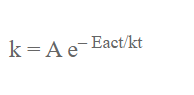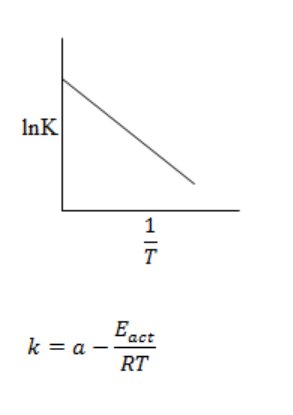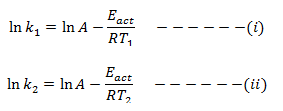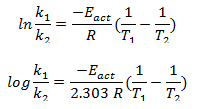# Arhenius Equation Dependence Of Rate On Temperature

The variation of rate or reaction on temperature is given by Arhenius equation. This eqn is:where,

k = Rate constant
Eact = Activation energy
R = Universal gas constant
T = Temperature in Kelvin
A = Constant called Arhenius factorIn a graph of lnk is plotted against 1/T it gives a straight line having y-intercept value lnA and slope -Eact/RT.

This shows that the rate constant is directly proportional to rate of reaction increase in temperature causes increase in rate of reaction.

If k1 and k2 are rate constant of a given reaction at T1 & T2.

Then,From equation (i) and (ii) we get,